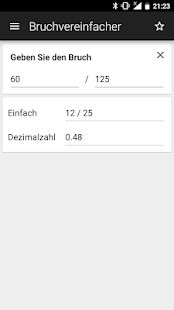# All-In-One Calculator v2.0.8 [Pro] [Mod] APK [Latest]All in one calculator pack, a must have for every Student and Enthusiast. Containing over 80 calculators and converters, it’s the only calculation tool box you’ll ever need on your device.

Large collection of Unit Converter, Electronics and Mathematics Calculators.

If you’re a scholar, student, teacher, enthusiast, engineer or handyman and you need an all in one calculator… CalcKit is the right calculation tool box for you.

Features:
★ Scientific Calculator
★ RPN Calculator (Reverse Polish notation)
★ Floating Scientific Calculator
★ The first ever Floating RPN Calculator for Android
★ 48 Mathematic Calculator Tools
★ 22 Electronic Calculator Tools
★ 14 Unit Converter
★ Custom Tools Creator
★ Supports Math Expressions as input
★ Tablet Support
★ 3 Calculator Themes
★ 5 Languages

Languages: English – French – German – Macedonian – Spanish

Feedbacks and suggestions
We’d love to hear from you!
Send us your feedbacks at ivan.gavrilov@yahoo.be

Uses permissions:
• Vibration (vibrate when button is clicked)
• Draw over other apps (CalcKit’s floating calculator)

Complete list of all calculator tools and unit converters in the Tool Box:

Scientific & RPN Calculator
Floating Scientific & RPN Calculator

Geometry
• Triangle
• Right Triangle
• Square
• Rectangle
• Parallelogram
• Rhombus
• Trapezoid
• Isosceles Trapezoid
• Regular Hexagon
• Regular Polygon
• Circle
• Segment of Circle
• Sector of Circle
• Ellipse
• Cube
• Cuboid
• Regular Prism
• Pyramid
• Pyramidal Frustum
• Regular Tetrahedron
• Regular Octahedron
• Cylinder
• Cylinder with an oblique plane face
• Cone
• Frustum of Cone
• Sphere
• Spherical Cap
• Spherical Sector
• Spherical Segment
• Spherical Wedge
• Torus

Equation
• Linear Equation
• Cubic Equation
• System 2×2
• System 3×3

Plane Analytic Geometry
• Distance between 2 points
• Area of Triangle
• Equation of Circle

Solid Analytic Geometry
• Distance between 2 points
• Equation of Sphere

Algebra
• Prime Number Check
• Proportion Calculator
• Decimal to Fraction
• Fraction Simplifier
• Prime Factorization
• GCF / LCM

Matrix Calculation
Electronics
• Resources – Resistivity Table
• Resources – Logic Gates
• Resources – Electronic Symbols
• Resistor Color Code
• Inductor Color Code
• LED Resistor Calculator
• Series Components
• Parallel Components
• Ohms Law
• Power Triangle
• Y – Δ Transformation
• Voltage Divider
• Voltage Regulator
• Operational Amplifier
• NE555 Timer
• Filters
• Reactance
• Wire Resistivity
• Transformer Ratio
• Battery Life Calculator
• Analog – Digital Converter
• Frequency Calculator

Unit Converter
• Numbers
• Digital Storage
• Length / Distance
• Mass / Weight
• Speed
• Area
• Volume
• Angle
• Energy / Work
• Fuel Consumption
• Power
• Temperature
• Time
• Pressure

Custom Tools Creator

What’s New:
✓ Added AWG Size Chart in Resources
✓ Some bug-fixes and translation improvements

If you have any questions or suggestions feel free to send an email at support@calckit.io at any time.

Mod Info:

Screenshots

••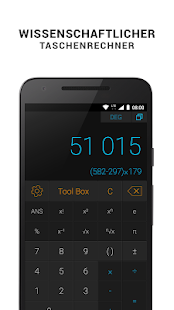•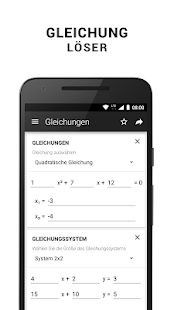•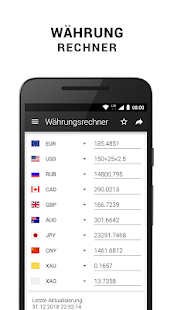•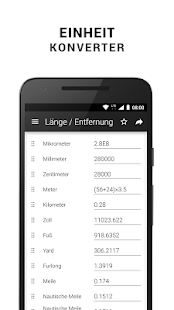•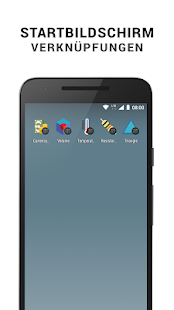•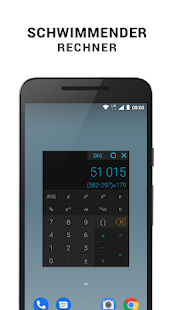•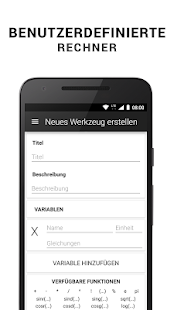•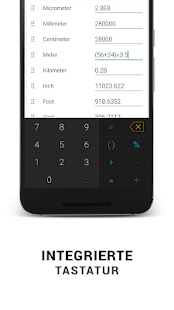•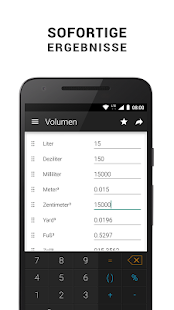•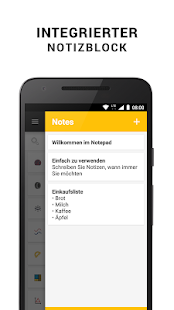•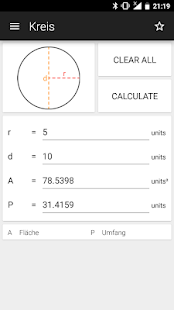•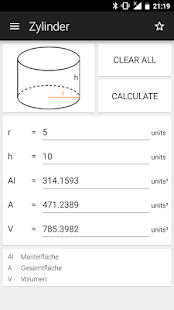••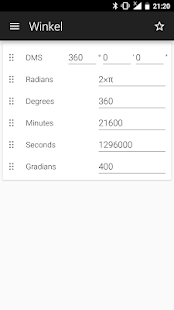•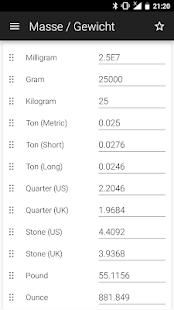•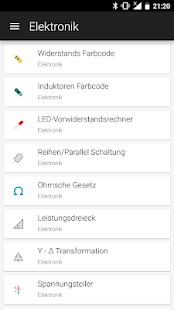•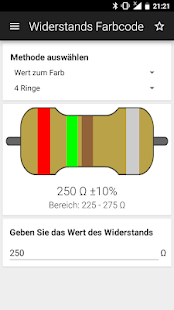•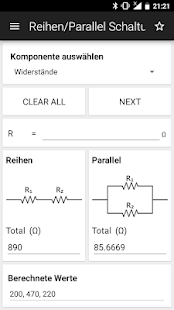•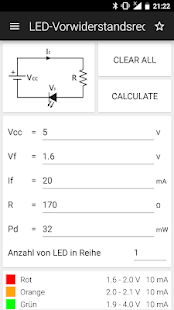•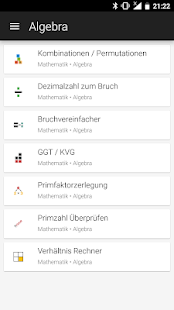•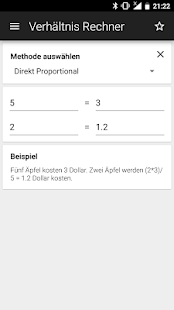•